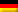### Transient heat transfer processes with LV

With WTS you can calculate classic shell-and-tube heat exchangers in which a shell-side medium is continuously cooled or heated. However, tube bundles can also be used to cool the contents of a tank in batch operation over a longer period of time. WTS also helps with this. This requires three steps:

1. Calculation of the inner heat transfer in WTS
2. Calculation of the outside heat transfer coefficient and the thermal transition
3. Calculation of the heating/cooling time with TIME

#### 1. Calculation of the inner heat transfer in WTS

First start the WTS module as usual. For a given coolant mass flow, WTS will help you determine a suitable number of tubes. The module also provides you with the properties of the fluid and calculates the heat transfer coefficient and the tube-side pressure drop. The prerequisite for this calculation is that fluid properties and heat transfer coefficients change only slightly in the area under consideration.

In the first selection screen, enter the tube diameter, pitch and tube material, as well as the fluids in the tube bundle and in the tank, and select a U-tube bundle. You can leave the other parameters at their initial values.

In the second screen, enter the start and end temperature of the tank medium as well as the coolant mass flow, its inlet temperature and an estimated outlet temperature. The WTS module now calculates a shell-and-tube heat exchanger.

The next step is to adjust the outside heat transfer coefficient. The resulting heat transfer coefficient is then used to calculate the cooling time.

#### 2. Calculation of the outside heat transfer coefficient and the thermal transition

In contrast to the shell-and-tube heat exchanger with forced convection, in this case there is a medium at rest. The heat transfer on the outside of the tube bundle is therefore mainly by free convection and is thus significantly lower than in the tube bundle heat exchanger.

In addition to WTS and the modules used here, the Heat programme also contains other modules for calculating heat transfer coefficients. Select module F2.1 for vertical cylinders here. This correlation was developed for single cylinders and therefore slightly overestimates the heat transfer coefficient for tube bundles. Therefore, plan a safety in your calculation. For information on the heat transfer coefficient in tube bundles, see e.g. .

For the calculation you need physical properties, which you can transfer to the input mask by dragging the corresponding properties module. You also need the tube diameter and length, as well as the temperature on the outside of the tube and of the medium in the exchanger. It is best to use the ” Link Variable” function for this, so that the calculation adjusts automatically in the event of changes.

The heat transfer coefficient calculated in this way can now be used to calculate the overall heat transfer coefficient. To do this, switch back to the WTS module. In the “Basic inputs” menu, click on “Specify alpha around the tubes”. This releases the outside heat transfer coefficient and you can connect it to the value calculated in F2.1. With inner and outer heat transfer coefficients as well as fouling, the overall heat transfer coefficient is now determined. Based on this, the cooling time is determined in the last step.

#### 3. Calculation of the cooling time with TIME

While a classic shell-and-tube heat exchanger is a steady-state process with constant temperatures, this case is transient. Over time, the medium in the tank approaches the coolant temperature and the transferred heat flow decreases. The time until cooling down to a given temperature can be calculated analytically with the help of the energy balance. Find this calculation in the TIME module.

Start the TIME module and select the option “Heating/cooling”. In the mask, enter the mass and heat capacity of the medium and tank. Then connect the cooling mass flow, its heat capacity and inlet temperature as well as the heat transfer surface and the overall heat transfer coefficient from WTS. This way you get the cooling time. Alternatively, you can also specify a cooling time, and thus calculate the required heat exchanger area, for example, to determine the required length of the tube bundle.

Dieser Beitrag ist auch verfügbar auf:Deutsch (German)